Notebook

The Correlation Coefficient¶

By Evgenia "Jenny" Nitishinskaya and Delaney Granizo-Mackenzie with example algorithms by David Edwards

Part of the Quantopian Lecture Series:

Notebook released under the Creative Commons Attribution 4.0 License. Please do not remove this attribution.

The correlation coefficient measures the extent to which the relationship between two variables is linear. Its value is always between -1 and 1. A positive coefficient indicates that the variables are directly related, i.e. when one increases the other one also increases. A negative coefficient indicates that the variables are inversely related, so that when one increases the other decreases. The closer to 0 the correlation coefficient is, the weaker the relationship between the variables.

The correlation coefficient of two series $X$ and $Y$ is defined as $$r = \frac{Cov(X,Y)}{std(X)std(Y)}$$ where $Cov$ is the covariance and $std$ is the standard deviation.

Two random sets of data will have a correlation coefficient close to 0:

Correlation vs. Covariance¶

Correlation is simply a normalized form of covariance. They are otherwise the same and are often used semi-interchangeably in everyday conversation. It is obviously important to be precise with language when discussing the two, but conceptually they are almost identical.

Covariance isn't that meaningful by itself¶

Let's say we have two variables $X$ and $Y$ and we take the covariance of the two.

In :
import numpy as np
import pandas as pd
import matplotlib.pyplot as plt
In :
X = np.random.rand(50)
Y = 2 * X + np.random.normal(0, 0.1, 50)

np.cov(X, Y)[0, 1]
Out:
0.14975887489776102

So now what? What does this mean? Correlation uses information about the variance of X and Y to normalize this metric. Once we've normalized the metric to the -1 to 1 scale, we can make meaningful statements and compare correlations.

To see how this is done consider the formula.

$$\frac{Cov(X, Y)}{std(X)std(Y)}$$$$= \frac{Cov(X, Y)}{\sqrt{var(X)}\sqrt{var(Y)}}$$$$= \frac{Cov(X, Y)}{\sqrt{Cov(X, X)}\sqrt{Cov(Y, Y)}}$$

To demonstrate this let's compare the correlation and covariance of two series.

In :
X = np.random.rand(50)
Y = 2 * X + 4

print 'Covariance of X and Y: \n' + str(np.cov(X, Y))
print 'Correlation of X and Y: \n' + str(np.corrcoef(X, Y))
Covariance of X and Y:
[[ 0.07228077  0.14456154]
[ 0.14456154  0.28912309]]
Correlation of X and Y:
[[ 1.  1.]
[ 1.  1.]]

Why do both np.cov and np.corrcoef return matricies?¶

The covariance matrix is an important concept in statistics. Often people will refer to the covariance of two variables $X$ and $Y$, but in reality that is just one entry in the covariance matrix of $X$ and $Y$. For each input variable we have one row and one column. The diagonal is just the variance of that variable, or $Cov(X, X)$, entries off the diagonal are covariances between different variables. The matrix is symmetric across the diagonal. Let's check that this is true.

In :
cov_matrix = np.cov(X, Y)

# We need to manually set the degrees of freedom on X to 1, as numpy defaults to 0 for variance
# This is usually fine, but will result in a slight mismatch as np.cov defaults to 1
error = cov_matrix[0, 0] - X.var(ddof=1)

print 'error: ' + str(error)
error: 1.38777878078e-17
In :
X = np.random.rand(50)
Y = np.random.rand(50)

plt.scatter(X,Y)
plt.xlabel('X Value')
plt.ylabel('Y Value')

# taking the relevant value from the matrix returned by np.cov
print 'Correlation: ' + str(np.cov(X,Y)[0,1]/(np.std(X)*np.std(Y)))
# Let's also use the builtin correlation function
print 'Built-in Correlation: ' + str(np.corrcoef(X, Y)[0, 1])
Correlation: 0.0612685087304
Built-in Correlation: 0.0600431385558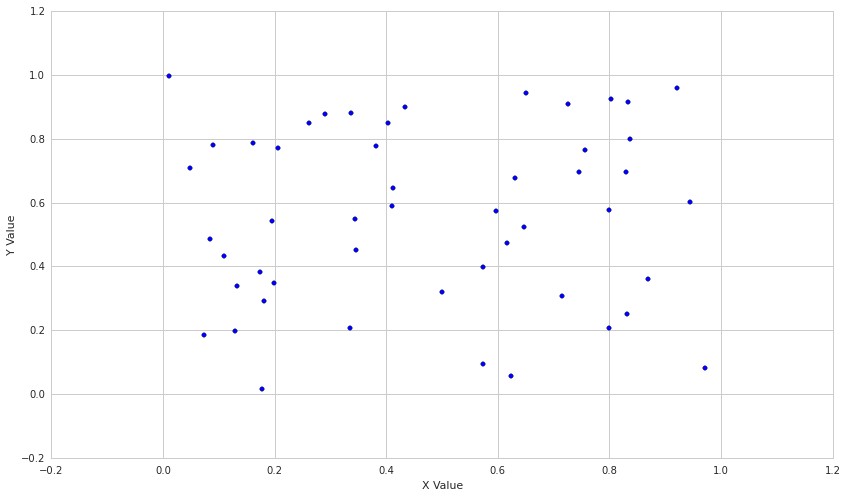Now let's see what two correlated sets of data look like.

In :
X = np.random.rand(50)
Y = X + np.random.normal(0, 0.1, 50)

plt.scatter(X,Y)
plt.xlabel('X Value')
plt.ylabel('Y Value')

print 'Correlation: ' + str(np.corrcoef(X, Y)[0, 1])
Correlation: 0.958613485688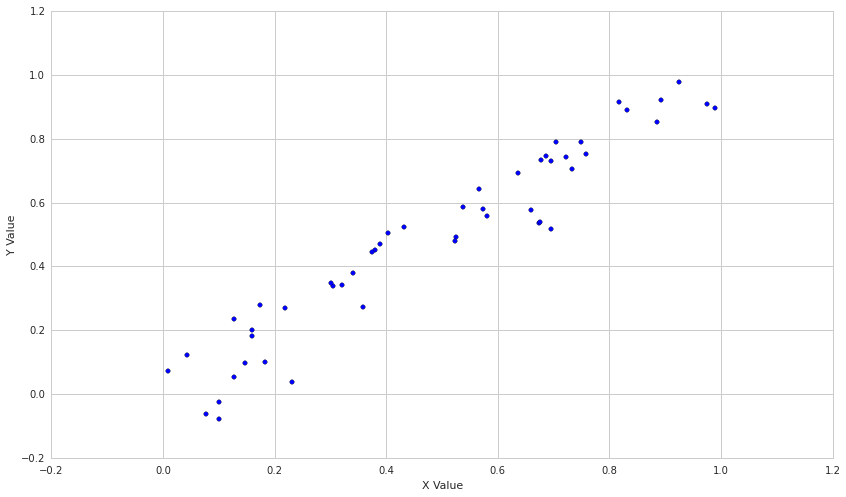Let's dial down the relationship by introducing more noise.

In :
X = np.random.rand(50)
Y = X + np.random.normal(0, .2, 50)

plt.scatter(X,Y)
plt.xlabel('X Value')
plt.ylabel('Y Value')

print 'Correlation: ' + str(np.corrcoef(X, Y)[0, 1])
Correlation: 0.879590392019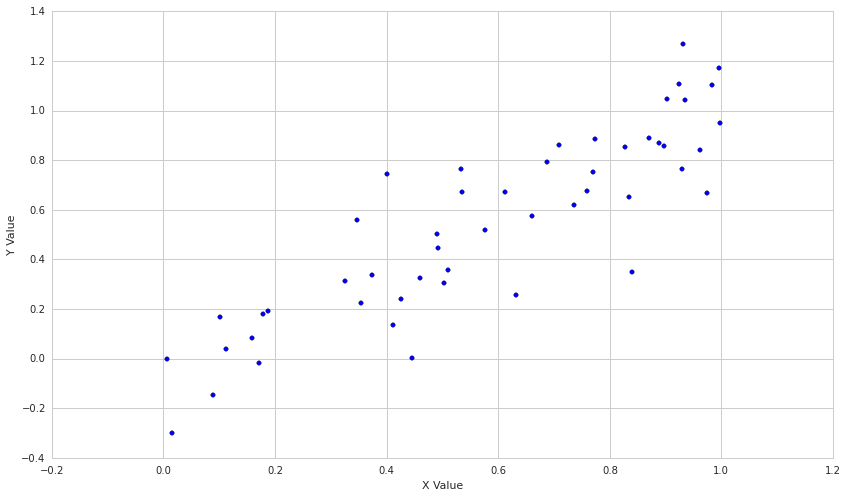Finally, let's see what an inverse relationship looks like.

In :
X = np.random.rand(50)
Y = -X + np.random.normal(0, .1, 50)

plt.scatter(X,Y)
plt.xlabel('X Value')
plt.ylabel('Y Value')

print 'Correlation: ' + str(np.corrcoef(X, Y)[0, 1])
Correlation: -0.944604531847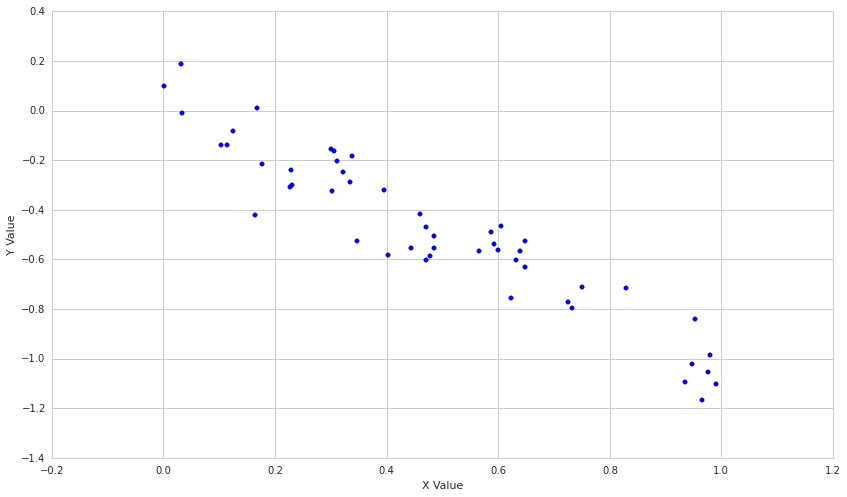We see a little bit of rounding error, but they are clearly the same value.

How is this useful in finance?¶

Once we've established that two series are probably related, we can use that in an effort to predict future values of the series. For example. let's loook at the price of Apple and a semiconductor equipment manufacturer, Lam Research Corporation.

In :
# Pull the pricing data for our two stocks and S&P 500
start = '2013-01-01'
end = '2015-01-01'
bench = get_pricing('SPY', fields='price', start_date=start, end_date=end)
a1 = get_pricing('LRCX', fields='price', start_date=start, end_date=end)
a2 = get_pricing('AAPL', fields='price', start_date=start, end_date=end)

plt.scatter(a1,a2)
plt.xlabel('LRCX')
plt.ylabel('AAPL')
plt.title('Stock prices from ' + start + ' to ' + end)
print "Correlation coefficients"
print "LRCX and AAPL: ", np.corrcoef(a1,a2)[0,1]
print "LRCX and SPY: ", np.corrcoef(a1,bench)[0,1]
print "AAPL and SPY: ", np.corrcoef(bench,a2)[0,1]
Correlation coefficients
LRCX and AAPL:  0.954684674528
LRCX and SPY:  0.935191172334
AAPL and SPY:  0.89214568707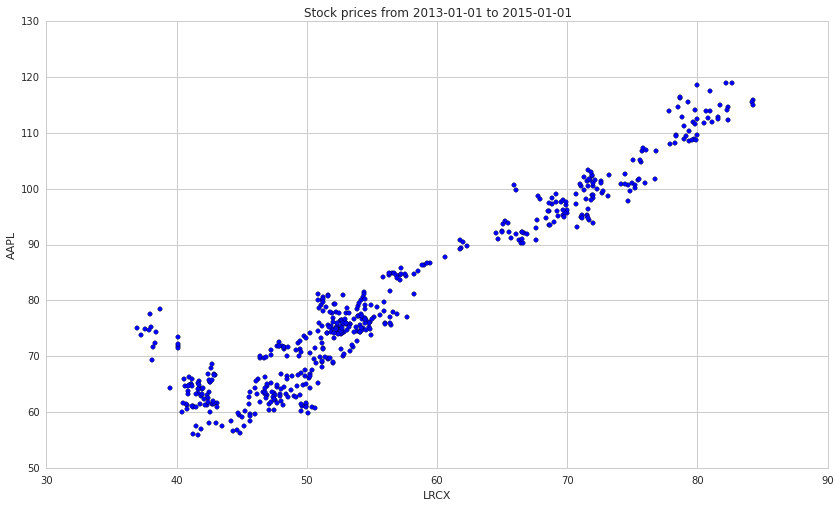Constructing a portfolio of uncorrelated assets¶

Another reason that correlation is useful in finance is that uncorrelated assets produce the best portfolios. The intuition for this is that if the assets are uncorrelated, a drawdown in one will not correspond with a drawdown in another. This leads to a very stable return stream when many uncorrelated assets are combined.

Limitations¶

Significance¶

It's hard to rigorously determine whether or not a correlation is significant, especially when, as here, the variables are not normally distributed. Their correlation coefficient is close to 1, so it's pretty safe to say that the two stock prices are correlated over the time period we use, but is this indicative of future correlation? If we examine the correlation of each of them with the S&P 500, we see that it is also quite high. So, AAPL and LRCX are slightly more correlated with each other than with the average stock.

One fundamental problem is that it is easy to datamine correlations by picking the right time period. To avoid this, one should compute the correlation of two quantities over many historical time periods and examine the distibution of the correlation coefficient. More details on why single point estimates are bad will be covered in future notebooks.

As an example, remember that the correlation of AAPL and LRCX from 2013-1-1 to 2015-1-1 was 0.95. Let's take the rolling 60 day correlation between the two to see how that varies.

In :
rolling_correlation = pd.rolling_corr(a1, a2, 60)
plt.plot(rolling_correlation)
plt.xlabel('Day')
plt.ylabel('60-day Rolling Correlation')
Out:
<matplotlib.text.Text at 0x7fe83920c610>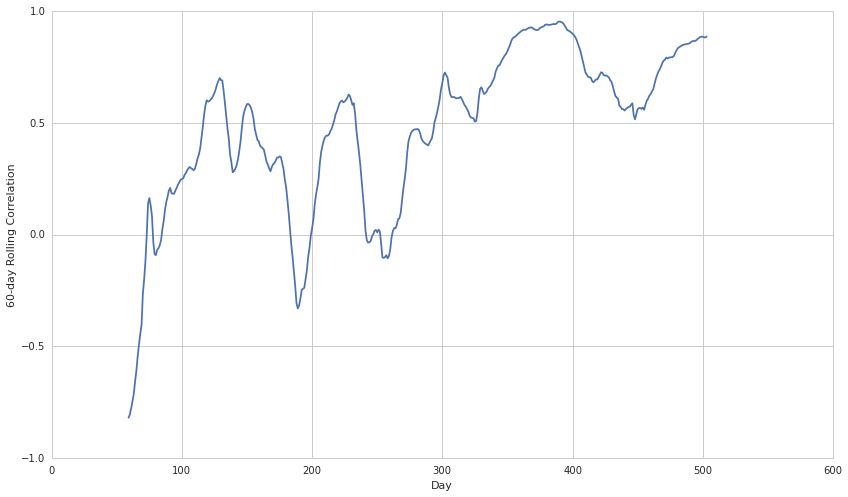Non-Linear Relationships¶

The correlation coefficient can be useful for examining the strength of the relationship between two variables. However, it's important to remember that two variables may be associated in different, predictable ways which this analysis would not pick up. For instance, one variable might precisely follow the behavior of a second, but with a delay. There are techniques for dealing with this lagged correlation. Alternatively, a variable may be related to the rate of change of another. Neither of these relationships are linear, but can be very useful if detected.

Additionally, the correlation coefficient can be very sensitive to outliers. This means that including or excluding even a couple of data points can alter your result, and it is not always clear whether these points contain information or are simply noise.

As an example, let's make the noise distribution poisson rather than normal and see what happens.

In :
X = np.random.rand(100)
Y = X + np.random.poisson(size=100)

plt.scatter(X, Y)

np.corrcoef(X, Y)[0, 1]
Out:
0.18485889539744632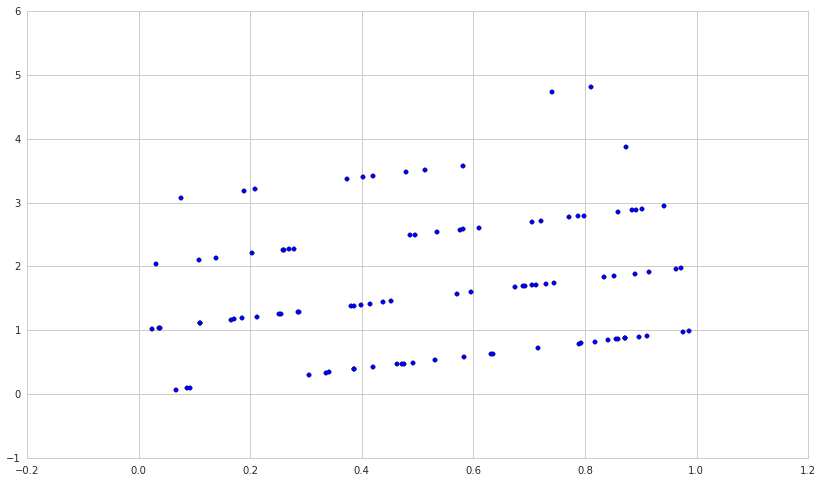In conclusion, correlation is a powerful technique, but as always in statistics, one should be careful not to interpret results where there are none.

This presentation is for informational purposes only and does not constitute an offer to sell, a solicitation to buy, or a recommendation for any security; nor does it constitute an offer to provide investment advisory or other services by Quantopian, Inc. ("Quantopian"). Nothing contained herein constitutes investment advice or offers any opinion with respect to the suitability of any security, and any views expressed herein should not be taken as advice to buy, sell, or hold any security or as an endorsement of any security or company. In preparing the information contained herein, Quantopian, Inc. has not taken into account the investment needs, objectives, and financial circumstances of any particular investor. Any views expressed and data illustrated herein were prepared based upon information, believed to be reliable, available to Quantopian, Inc. at the time of publication. Quantopian makes no guarantees as to their accuracy or completeness. All information is subject to change and may quickly become unreliable for various reasons, including changes in market conditions or economic circumstances.